Share

# NCERT solutions for Class 9 Mathematics Textbook chapter 8 - Quadrilaterals [Latest edition]

Course
Textbook page

#### Chapters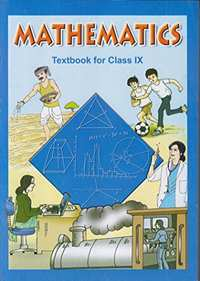Ex. 8.1Ex. 8.2

#### NCERT solutions for Class 9 Mathematics Textbook Chapter 8 QuadrilateralsExercise 8.1 [Pages 146 - 147]

Ex. 8.1 | Q 1 | Page 146

The angles of quadrilateral are in the ratio 3: 5: 9: 13. Find all the angles of the quadrilateral.

Ex. 8.1 | Q 2 | Page 146

If the diagonals of a parallelogram are equal, then show that it is a rectangle.

Ex. 8.1 | Q 3 | Page 146

Show that if the diagonals of a quadrilateral bisect each other at right angles, then it is a rhombus.

Ex. 8.1 | Q 4 | Page 146

Show that the diagonals of a square are equal and bisect each other at right angles.

Ex. 8.1 | Q 5 | Page 146

Show that if the diagonals of a quadrilateral are equal and bisect each other at right angles, then it is a square.

Ex. 8.1 | Q 6 | Page 146

Diagonal AC of a parallelogram ABCD bisects ∠A (see the given figure). Show that

(i) It bisects ∠C also,

(ii) ABCD is a rhombus.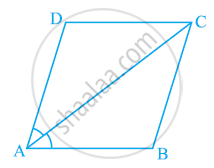Ex. 8.1 | Q 7 | Page 146

ABCD is a rhombus. Show that diagonal AC bisects ∠A as well as ∠C and diagonal BD bisects ∠B as well as ∠D.

Ex. 8.1 | Q 8 | Page 146

ABCD is a rectangle in which diagonal AC bisects ∠A as well as ∠C. Show that:-

(i) ABCD is a square (ii) diagonal BD bisects ∠B as well as ∠D.

Ex. 8.1 | Q 9 | Page 147

In parallelogram ABCD, two points P and Q are taken on diagonal BD such that DP = BQ (see the given figure). Show that:

(i) ΔAPD ≅ ΔCQB

(ii) AP = CQ

(iii) ΔAQB ≅ ΔCPD

(iv) AQ = CP

(v) APCQ is a parallelogram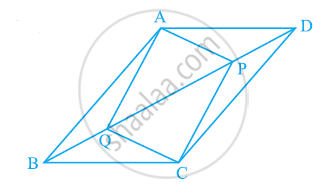Ex. 8.1 | Q 10 | Page 147

ABCD is a parallelogram and AP and CQ are perpendiculars from vertices A and C on diagonal BD (See the given figure). Show that

(i) ΔAPB ≅ ΔCQD

(ii) AP = CQ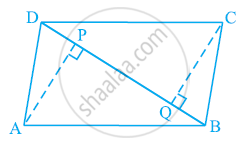Ex. 8.1 | Q 11 | Page 147

In ΔABC and ΔDEF, AB = DE, AB || DE, BC = EF and BC || EF. Vertices A, B and C are joined to vertices D, E and F respectively (see the given figure). Show that

(i) Quadrilateral ABED is a parallelogram

(ii) Quadrilateral BEFC is a parallelogram

(iv) Quadrilateral ACFD is a parallelogram

(v) AC = DF

(vi) ΔABC ≅ ΔDEF.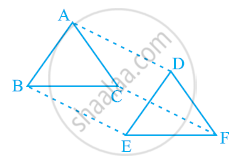Ex. 8.1 | Q 12 | Page 147

ABCD is a trapezium in which AB || CD and AD = BC (see the given figure). Show that

(i) ∠A = ∠B

(ii) ∠C = ∠D

(iv) diagonal AC = diagonal BD

[Hint: Extend AB and draw a line through C parallel to DA intersecting AB produced at E.]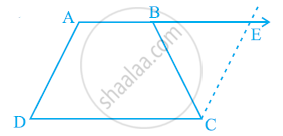#### NCERT solutions for Class 9 Mathematics Textbook Chapter 8 QuadrilateralsExercise 8.2 [Pages 150 - 151]

Ex. 8.2 | Q 1 | Page 150

ABCD is a quadrilateral in which P, Q, R and S are mid-points of the sides AB, BC, CD and DA (see the given figure). AC is a diagonal. Show that:

(i) SR || AC and SR = 1/2AC

(ii) PQ = SR

(iii) PQRS is a parallelogram.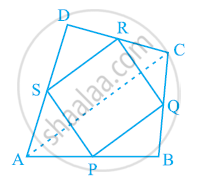Ex. 8.2 | Q 2 | Page 150

ABCD is a rhombus and P, Q, R and S are the mid-points of the sides AB, BC, CD and DA respectively. Show that the quadrilateral PQRS is a rectangle.

Ex. 8.2 | Q 3 | Page 150

ABCD is a rectangle and P, Q, R and S are mid-points of the sides AB, BC, CD and DA respectively. Show that the quadrilateral PQRS is a rhombus.

Ex. 8.2 | Q 4 | Page 150

ABCD is a trapezium in which AB || DC, BD is a diagonal and E is the mid - point of AD. A line is drawn through E parallel to AB intersecting BC at F (see the given figure). Show that F is the mid-point of BC.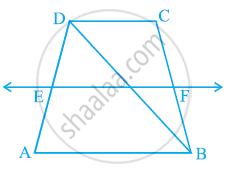Ex. 8.2 | Q 5 | Page 151

In a parallelogrFind the values of k for each of the following quadratic equations, so that they have two equal roots. 2x2 + kx + 3 = 0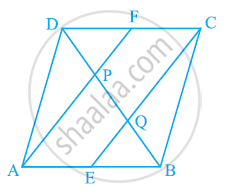Ex. 8.2 | Q 6 | Page 151

Show that the line segments joining the mid-points of the opposite sides of a quadrilateral bisect each other.

Ex. 8.2 | Q 7 | Page 151

ABC is a triangle right angled at C. A line through the mid-point M of hypotenuse AB and parallel to BC intersects AC at D. Show that

(i) D is the mid-point of AC

(ii) MD ⊥ AC

(iii) CM = MA = 1/2AB

Ex. 8.1Ex. 8.2## NCERT solutions for Class 9 Mathematics Textbook chapter 8 - Quadrilaterals

NCERT solutions for Class 9 Mathematics Textbook chapter 8 (Quadrilaterals) include all questions with solution and detail explanation. This will clear students doubts about any question and improve application skills while preparing for board exams. The detailed, step-by-step solutions will help you understand the concepts better and clear your confusions, if any. Shaalaa.com has the CBSE Class 9 Mathematics Textbook solutions in a manner that help students grasp basic concepts better and faster.

Further, we at Shaalaa.com provide such solutions so that students can prepare for written exams. NCERT textbook solutions can be a core help for self-study and acts as a perfect self-help guidance for students.

Concepts covered in Class 9 Mathematics Textbook chapter 8 Quadrilaterals are Concept of Quadrilaterals, Angle Sum Property of a Quadrilateral, Types of Quadrilaterals, Another Condition for a Quadrilateral to Be a Parallelogram, The Mid-point Theorem, Theorem : a Diagonal of a Parallelogram Divides It into Two Congruent Triangles, Theorem : a Diagonal of a Parallelogram Divides It into Two Congruent Triangles, In a Parallelogram, Opposite Sides Are Equal. Ab = Cd and Bc = Da, Theorem : If Each Pair of Opposite Sides of a Quadrilateral is Equal, Then It is a Parallelogram., In a Parallelogram, Opposite Angles Are Equal., Theorem: If in a Quadrilateral, Each Pair of Opposite Angles is Equal, Then It is a Parallelogram., The Diagonals of a Parallelogram Bisect Each Other., Theorem : If the Diagonals of a Quadrilateral Bisect Each Other, Then It is a Parallelogram.

Using NCERT Class 9 solutions Quadrilaterals exercise by students are an easy way to prepare for the exams, as they involve solutions arranged chapter-wise also page wise. The questions involved in NCERT Solutions are important questions that can be asked in the final exam. Maximum students of CBSE Class 9 prefer NCERT Textbook Solutions to score more in exam.

Get the free view of chapter 8 Quadrilaterals Class 9 extra questions for Class 9 Mathematics Textbook and can use Shaalaa.com to keep it handy for your exam preparation

S